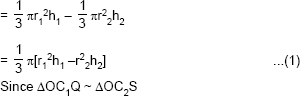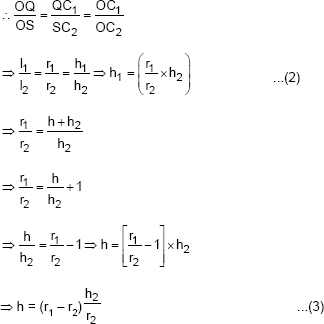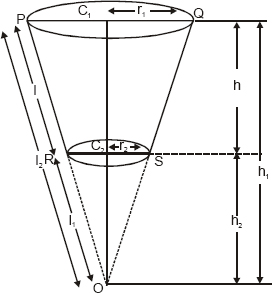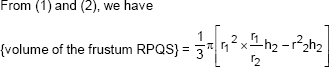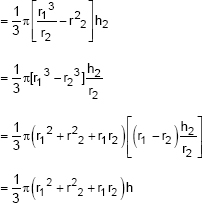# Surface Areas and Volumes-NCERT Solutions

Class X Math
NCERT Solution for Surface Areas and Volumes
Exercise 13.1
Q.1.   Two cubes each of volume 64 cm3 are joined end to end. Find the surface area of the resulting cuboid.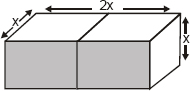Sol. Volume of each cube = 64 cm3
∴ Total volume of the two cubes = 2 × 64 cm3
= 128 cm3
Let the edge of each cube = x
∴ x3 = 64 = 43
∴ x = 4 cm
Now, Length of the resulting cuboid l = 2x cm
Breadth of the resulting cuboid b = x cm
Height of the resulting cuboid h = x cm
∴ Surface area of the cuboid = 2 (lb + bh + hl) = 2[(2x . x) + (x . x) + (x . 2x)]
= 2[(2 × 4 × 4) + (4 × 4) + (4 × 2 × 4)] cm2
= 2 [32 + 16 + 32] cm2 = 2 cm2 = 160 cm2.
Q.2.   A vessel is in the form of a hollow hemisphere mounted by a hollow cylinder. The diameter of the hemisphere is 14 cm and the total height of the vessel is 13 cm. Find the inner surface area of the vessel.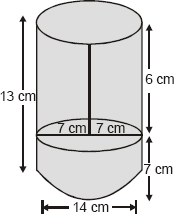Sol. For cylindrical part:
Height (h) = 6 cm
∴ Curved surface area
= 2πrh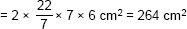For hemispherical part:
∴ Surface area = 2πr2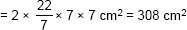∴ Total surface area
= (264 + 308) cm2 = 572 cm2.
Q.3.   A toy is in the form of a cone of radius 3.5 cm mounted on a hemisphere of same radius. The total height of the toy is 15.5 cm. Find the total surface area of the toy.
Sol. Here, r = 3.5 cm
∴ h = (15.5 – 3.5) cm = 12.0 cm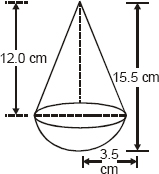Surface area of the conical part
= πrl
Surface area of the hemispherical part
= 2πr2
∴ Total surface area of the toy
= πrl + 2πr2 = πr (l + 2r) cm2
∵ l2 = (12)2 + (3.5)2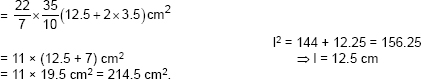Q.4.   A cubical block of side 7 cm is surmounted by a hemisphere. What is the greatest diameter the hemisphere can have? Find the surface area of the solid.
Sol. Side of the block = 7 cm
⇒ The greatest diameter of the hemisphere = 7 cm
Surface area of the solid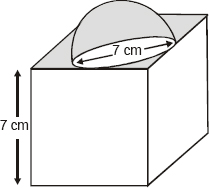= [Total S.A. of the cubical block] + [S.A. of the hemisphere] – [Base area of the hemisphere]
= (6 × l2) + 2πr2 – πr2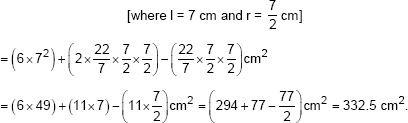Q.5.   A hemispherical depression is cut out from one face of a cubical wooden block such that the diameter l of the hemisphere is equal to the edge of the cube. Determine the surface area of the remaining solid.
Sol. Let ‘l’ be the side of the cube.
∴ The greatest diameter of the curved hemisphere = l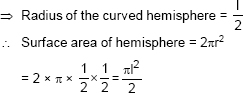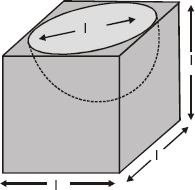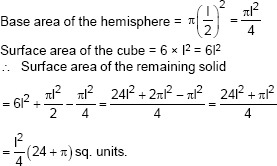Q.6.   A medicine capsule is in the shape of a cylinder with two hemispheres stuck to each of its ends (see Fig.). The length of the entire capsule is 14 mm and the diameter of the capsule is 5 mm. Find its surface area.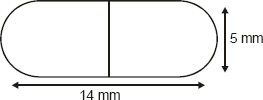Sol. Radius of the hemispherical part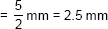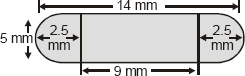∴ Surface area of one hemispherical part = 2πr2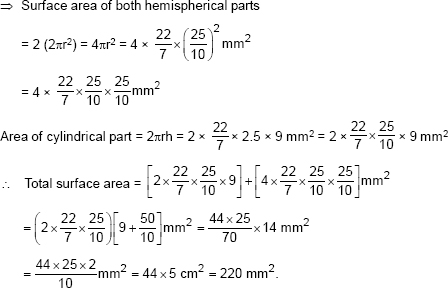Q.7.   A tent is in the shape of a cylinder surmounted by a conical top. If the height and diameter of the cylindrical part are 2.1 m and 4 m respectively, and the slant height of the top is 2.8 m, find the area of the canvas used for making the tent. Also, find the cost of the canvas of the tent at the rate of Rs. 500 per m2. (Note that the base of the will not be covered with canvas.)
Sol. For cylindrical part: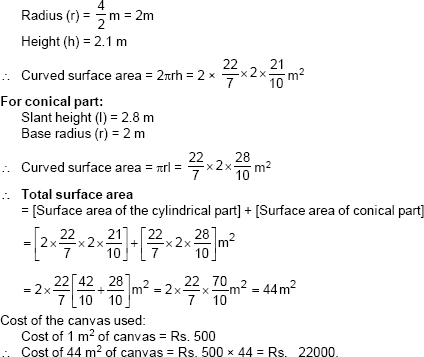Q.8.   From a solid cylinder whose height is 2.4 cm and diameter 1.4 cm, a conical cavity of the same height and same diameter is hollowed out. Find the total surface area of the remaining solid to the nearest cm2.
Sol. For cylindrical part:
Height = 2.4 cm
Diameter = 1.4 cm
⇒ Radius (r) = 0.7 cm
⇒ Total surface area of the cylindrical part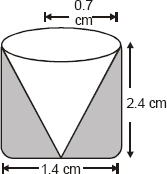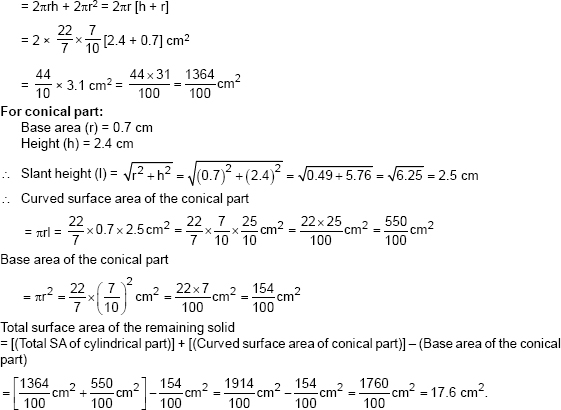Q.9.   A wooden article was made by scooping out a hemisphere from each end of a solid cylinder, as show in Fig. If the height of the cylinder is 10 cm, and its base is of radius 3.5 cm, find the total surface area of the article.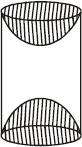Sol. Radius of the cylinder (r) = 3.5 cm
Height of the cylinder (h) = 10 cm
∴ Total surface area = 2πrh + 2πr2 = 2πr(h +r)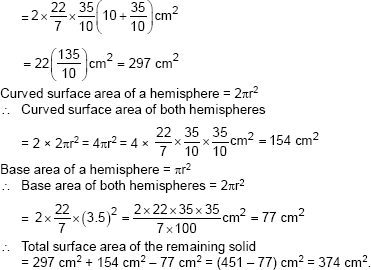Exercise 13.2
Q.1.   A solid is in the shape of a cone standing on a hemisphere with both their radii being equal to 1 cm and the height of the cone is equal to its radius. Find the volume of the solid in terms of π.
Sol. Here, r = 1 cm and h = 1 cm.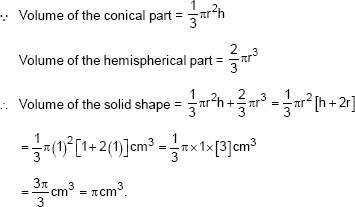Q.2.   Rachel, an engineering student, was asked to make a model shaped like a cylinder with two cones attached at its two ends by using a thin aluminium sheet. The diameter of the model is 3 cm and its length is 12 cm. If each cone has a height of 2 cm, find the volume of air contained in the model that Rachel made. (Assume the outer and inner dimensions of the model to be nearly the same.)
Sol. Here, diameter = 3 cm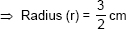Total height = 12 cm
Height of a cone (h1) = 2 cm
∴ Height of both cones = 2 × 2 = 4 cm
⇒ Height of the cylinder (h2) = (12 – 4) cm = 8 cm.
Now, volume of the cylindrical part = πr2h2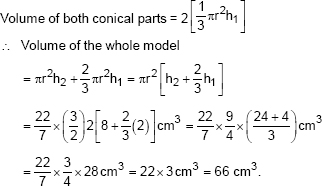Q.3.   A gulab jamun, contains sugar syrup up to about 30% of its volume. Find approximately how much syrup would be found in 45 gulabl jamuns, each shaped like a cylinder with two hemispherical ends with length 5 cm and diameter 2.8 cm (see figure).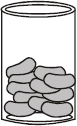Sol. Since, a gulab jamun is like a cylinder with hemispherical ends.
Total height of the gulab jamun = 5 cm.
Diameter = 2.8 cm
∴ Length (height) of the cylindrical part = 5 cm – (1.4 + 1.4) cm
= 5 cm – 2.8 cm =2.2 cm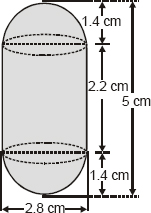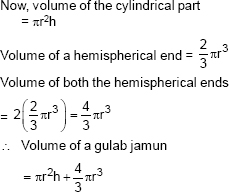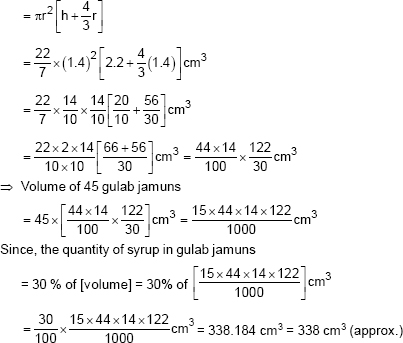Q.4.   A pen stand made of wood is in the shape of a cuboid with four conical depressions to hold pens. The dimensions of the cuboid are 15 cm by 10 cm by 3.5 cm. The radius of each of the depressions is 0.5 cm and the depth is 1.4 cm. Find the volume of wood in the entire stand (see Fig.).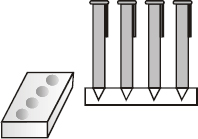Sol. Dimensions of the cuboid are 15 cm, 10 cm and 3.5 cm.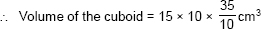= 15 × 35 cm3
= 525 cm3
Since each depression is conical with base radius (r) = 0.5 cm and depth (h) = 1.4 cm,
∴ Volume of each depression (cone)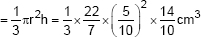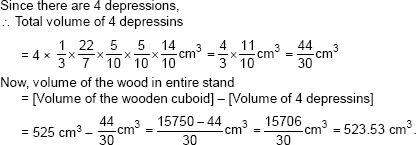Q.5.   A vessel is in the form of an inverted cone. its height is 8 cm and the radius of its top, which is open, is 5 cm. It is filled with water up to the brim. When lead shots, each of which is a sphere of radius 0.5 cm are dropped into the vessel, one-forth of the water flows out. Find the number of lead shots dropped in the vessel.
Sol. Height of the conical vessel (h) = 8 cm
Base radius (r) = 5 cm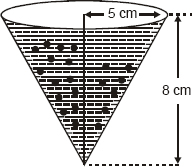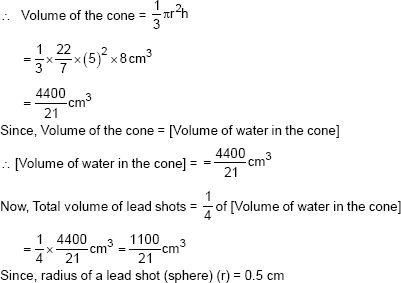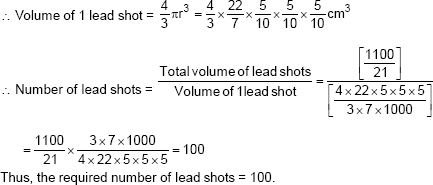Q.6.   A solid iron pole consists of a cylinder of height 220 cm and base diameter 24 cm, which is surmounted by another cylinder of height 60 cm and radius 8 cm. Find the mass of the pole, given that 1 cm3 of iron has approximately 8 g mass.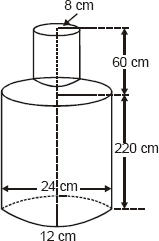Sol. Height of the big cylinder (h) = 220 cm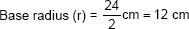∴ Volume of the big cylinder = pr2h = π(12)2 × 220 cm3
Also, height of smaller cylinder (h1) = 60 cm
Base radius (r1) = 8 cm
∴ Volume of the smaller cylinder πr12h1 = π(8)2 × 60 cm3
∴ Volume of iron
= [Volume of big cylinder] + [Volume of the smaller cylinder]
= π × 220 × 122 + π × 60 × 82 cm3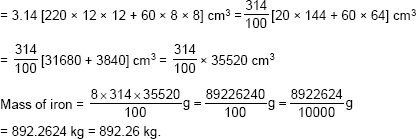Q.7.   A solid consisting of a right circular cone of height 120 cm and radius 60 cm standing on a hemisphere of radius 60 cm is placed upright in a right circular cylinder full of water such that it touches the bottom. Find the volume of water left in the cylinder, if the radius of the cylinder is 60 cn and its height is 180 cm.
Sol. Height of the conical part = 120 cm.
Base radius of the conical part = 60 cm.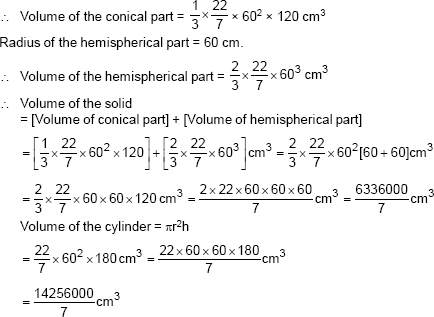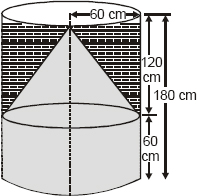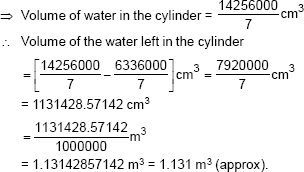Q.8.   A spherical glass vessel has a cylindrical neck 8 cm long, 2 cm in diameter; the diameter of the spherical part is 8.5 cm. By measuring the amount of water it holds, a child finds its volume to be 345 cm3. Check whether she is correct, taking the above as the inside measurements, and π = 3.14.
Sol. Volume of the cylindrical part
= πr2h = 3.14 × 12 × 8 cm3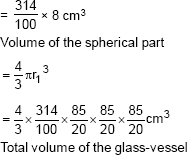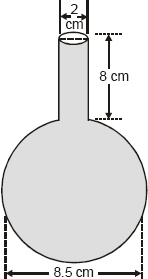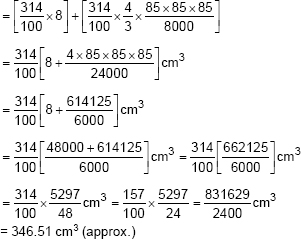⇒ Volume of water in the vessel = 346.51 cm3
Since, the child finds the volume as 345 cm3
∴ The child’s answer is not correct
⇒ The correct answer is 346.51 cm3.
Exercise 13.3
Q.1.   A metallie sphere of radius 4.2 cm is melted and recast into the shape of a cylinder of radius 6 cm. Find the height of the cylinder.
Sol. Radius of the sphere (r1) = 4.2 cm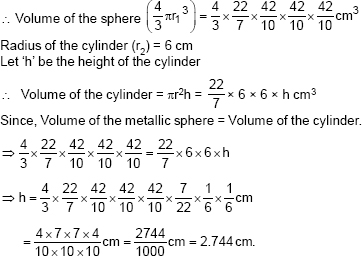Q.2.   Metallic spheres of radii 6 cm, 8 cm and 10 cm, respectively, are melted to form a single solid sphere. Find the radius of the resulting sphere.
Sol. Radii of the given spheres are:
r1 = 6 cm
r2 = 8 cm
r3 = 10 cm
⇒ Volume of the given spheres are: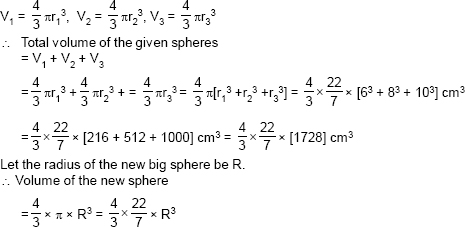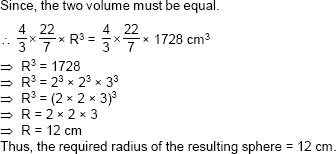Q.3.   A 20 m deep well with diameter 7 m is dug and the earth from digging is evenly spread out to form a platform 22 m by 14 m. Find the height of the platform.
Sol. Diameter of the cylindrical well = 7 m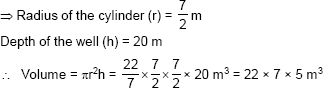⇒ Volume of the earth taken out = 22 × 7 × 5 m3
Now this earth is spread out to form a cuboial platform having
length = 22 m
Let ‘h’ be the height of the platform.
∴ Volume of the platform = 22 × 14 × h m3
∴ 22 × 14 × h = 22 × 7 × 5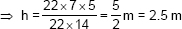Thus, the required height of the platform is 2.5 m.
Q.4.   A well of diamter 3 m is dug 14 m deep. The earth taken out of it has been spread evenly all around it in shape of a circular ring of width 4 m to form an embankment. Find the height of the embankment.
Sol. Diameter of cylindrical well (d) = 3 m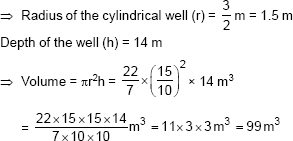Let the height of the embankment = ‘H’ metre.
Internal radius of the embankment (r) = 1.5 m.
External radius of the embankment R = (4 + 1.5)m = 5.5 m.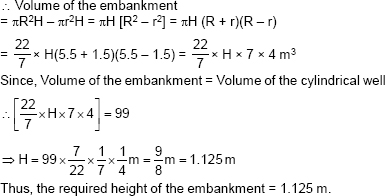Q.5.   A container shaped like a right circular cylinder having diameter 12 cm and height 15 cm is full of ice cream. The ice cream is to be filled into cones of height 12 cm and diameter 6 cm, having a hemispherical shape on the top. Find the number of such cones which can be filled with ice cream.
Sol. For the circular cylinder: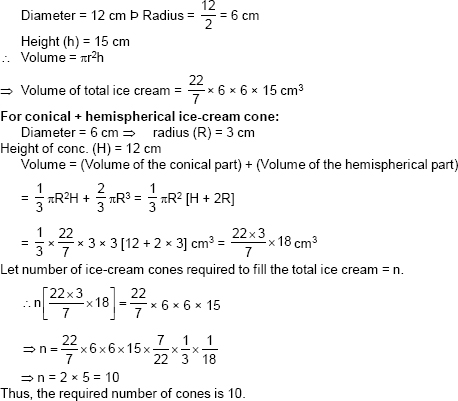Q.6.   How many silver coins, 1.75 cm in diameter and of thickness 2 mm, must be melted to form a cuboid of dimensions 5.5 cn × 10 cm × 3.5 cm?
Sol. For a circular coin: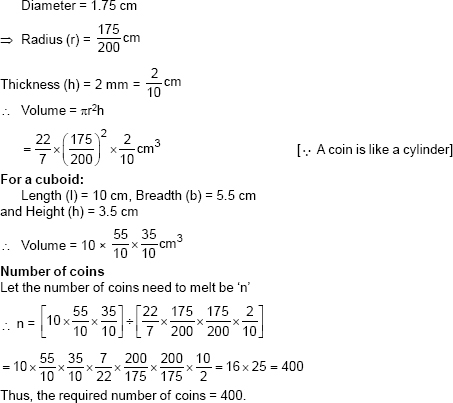Q.7.   A cylindrical bucket, 32 cm high and with radius of base 18 cm, is filled with sand. This bucket is emptied on the ground and a conical heap of sand is formed. If the height of the conical heap is 24 cm, find the radius and slant height of the heap.
Sol. For the cylindrical bucket:
Height (h) = 32 cm
Volume = πr2h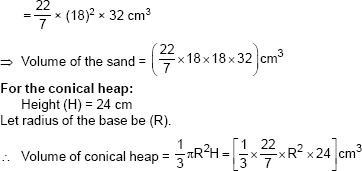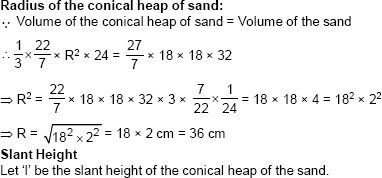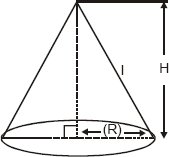Q.8.   Water in a canal, 6 m wide and 1.5 m deep, is flowing with a speed of 10 km/h. How much area will it irrigate in 30 minutes, if 8 cm of standing water is needed?
Sol. Width of the canal = 6 m
Depth of the canal = 1.5 m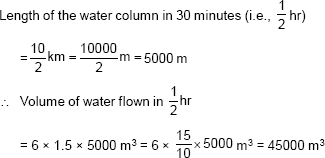Since the above amount (volume) of water is spread in the form of a cuboid of height as 8 cm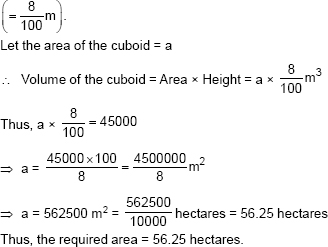Q.9.   A farmer connects a pipe of internal diameter 20 cm from a canal into a cylindrical tank in her field, which is 10 m in diameter and 2 m deep. If water flows through the pipe at the rate of 3 km/h, in how much time will the tank be filled?
Sol. Diameter of the pipe = 20 cm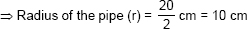Since, the water flows through the pipe at 3 km/hr.
∴ Length of water column per hour
(h) = 3 km = 3 × 1000 m = 3000 × 100 cm = 300000 cm.
∴ Volume of water = πr2h = π × 102 × 300000 cm3 = π × 30000000 cm3
Now, for the cylindrical tank,
Diameter = 10 m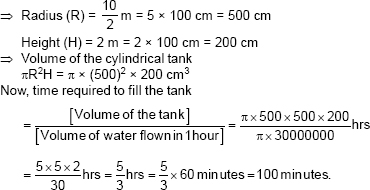Exercise 13.4
Q.1.   A drinking glass is in the shape of a frustum of a cone of height 14 cm. The diameters of its two circular ends are 4 cm and 2 cm. Find the capacity of the glass.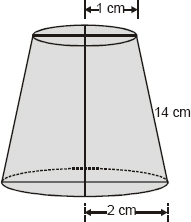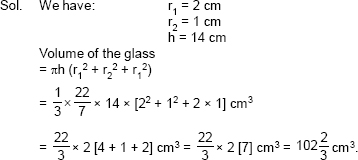Q.2.   The slant height of a frustum of a cone is 4 cm and the perimeters (circumference) of its circular ends are 18 cm and 6 cm. Find the surface area of the frustum.
Sol. We have:
Slant height (l) = 4 cm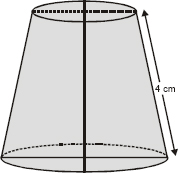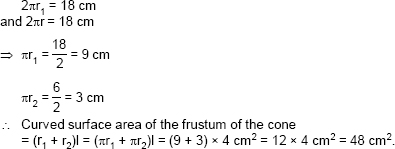Q.3.   A fez, the cap used by the Turks, is shaped like the frustum of a cone (see Fig.). If its radius on the open side is 10 cm, radius at the upper base is 4 cm and its slant height is 15 cm, find the area of material used for making it.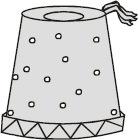Sol. Here, the radius of the open side (r1) = 10 cm
The radius of the upper base (r2) = 4 cm
Slant height (l) = 15 cm
∴ Area of the material required
= [Curved surface area of the frustum] + [Area of the top end]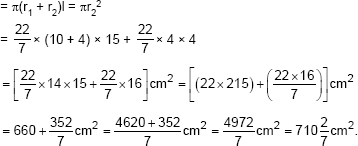Q.4.   A container, opened from the top and made up of a metal sheet, is in the form of a frustum of a cone of height 16 cm with radii of its lower and upper ends as 8 cm and 20 cm, respectively. Find the cost of the milk which can completely fill the container, at the rate of Rs. 20 per litre. Also find the cost of metal sheet used to make the container, if it costs Rs. 8 per 100 cm2.
Sol. We have:                                                  r1 = 20 cm, r2 = 8 cm
and                                                                h = 16 cm
∴ Volume of the frustum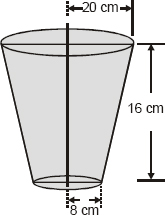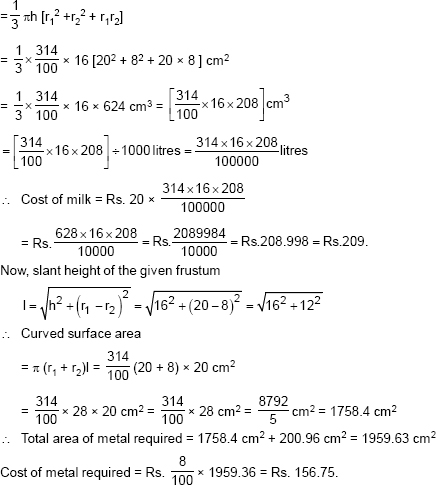Q.5.   A metallic right circular cone 20 cm high and whose vertical angle is 60° is cut into two parts at the middle of its height by a plane parallel to its base. If the frustum so obtained be drawn into a wire of diameter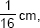find the length of the wire.
Sol. Let us consider the frustum DECB of the metallic cone ABC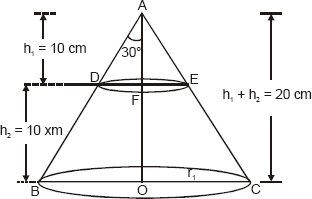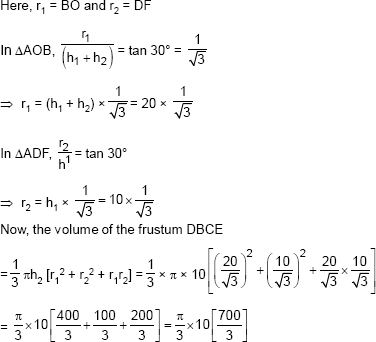Let l be the length and D be diameter of the wire drawn from the frustum. Since the wire is in the form of a cylinder,
∴ Volume of the wire = πr2l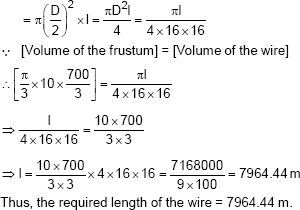Exercise 13.5
Q.1.   A copper wire, 3 mm in diameter, is wound about a cylinder whose length is 12 cm, and diameter 10 cm, so as to cover the curved surface of the cylinder. Find the length and mass of the wire, assuming the density of copper to be 8.88 g per cm3.
Sol. Since, diameter of the cylinder = 10 cm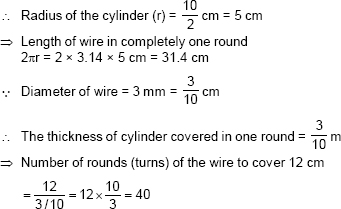∴ Length of wire required to cover the whole surface = Length of wire required to complete 40 rounds
= 40 × 31.4 cm = 1256 cm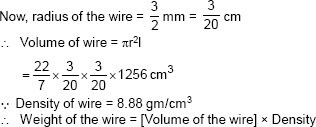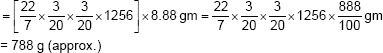Q.2.   A right triangle, whose sides are 3 cm and 4 cm (other than hypotenuse) is made to revolve about its hypotenuse. Find the volume and surface area of the double cone so formed. Choose value of π as found appropriate.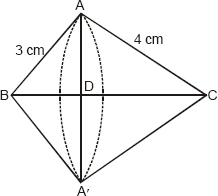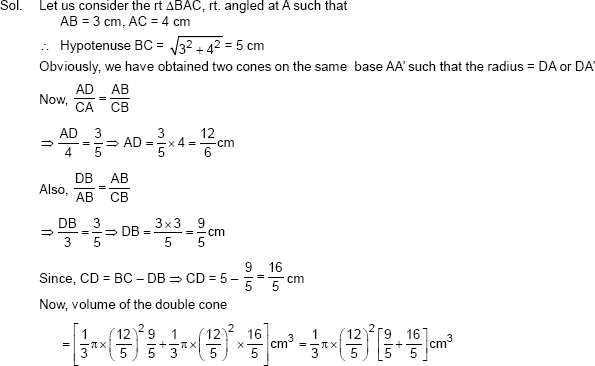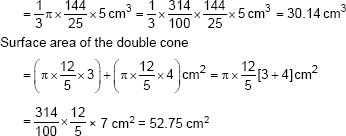Q.3.   A cistern, internally measuring 150 cm × 120 cm × 100 cm, has 129600 cm3 of water in it. Porous bricks are placed in the water until the cistern is full to the brim. Each brick absorbs one-seventeenth of its own volume of water. How many bricks can be put in without ocerflowing the water, each being 22.5 cm × 7.5 cm × 6.5 cm?
Sol. ∵ Dimensions of the cistern are 150 cm, 120 cm and 110 cm.
∴ Volume of the cistern = 150 × 120 × 110 cm3 = 1980000 cm3
Volume of water contained in the cistern = 129600 cm3
∴ Free space (volume) which is not filled with water
= 1980000 – 129600 cm3 = 1850400 cm3
Now, Volume of one brick = 22.5 × 7.5 × 6.5 cm3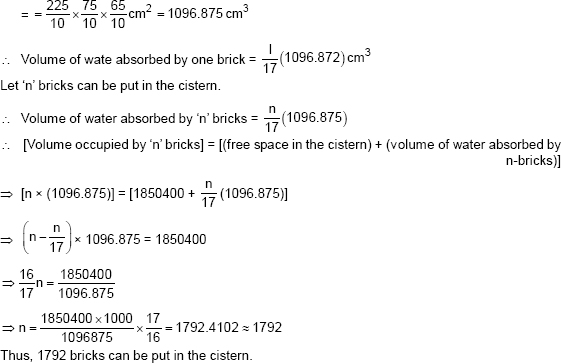Q.4.   In one fortnight of a given month, there was a rainfall of 10 cm in a river valley. If the area of the valley is 97280 km2, show that the total rainfall was approximately equivalent to the addition to the normal water of three rivers each 1072 km long, 75 m wide and 3 m deep.
Sol. Volume of three rivers = 3 {(Surface area of a river) × Depth}Q.5.   An oil funnel made of tin sheet consists of a 10 cm long cylindrical portion attached to a frustum of a cone. If the total height is 22 cm, diameter of the cylindrical portion is 8 cm and the diameter of the top of the funnel is 18 cm, find the area of the tin sheet required to make the funnel (see Fig.).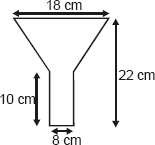Sol. We have, for the cylindrical part
Diameter = 8 cm
⇒ Radius (r) = 4 cm
Height = 10 cm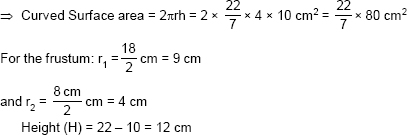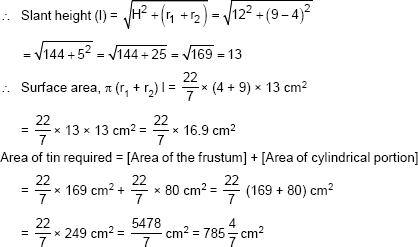Q.6.   Derive the formula for the curved surface area and total surface area of the frustum of a cone, given to you in Section 13.5, using the symbols as explained.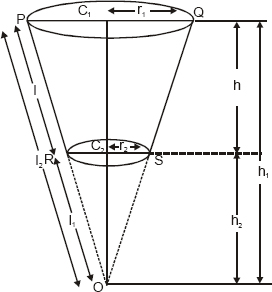Sol. We have,
Curved surface area of the frustum PQRS
= [curved surface area of the rt circular cone OPQ] – [curved surface area of the rt circular cone ORS]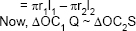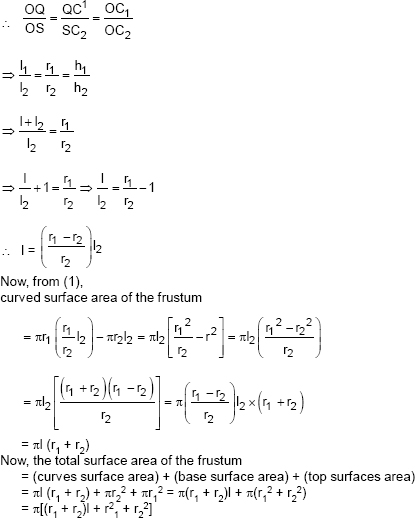Q.7.   Derive the formula for the volume of the frustum of a cone, given to you in Section 13.5, using the symbols as explained.
Sol. We have,
[Volume of the frustum RPQS]
= [Volume of right circular cone OPQ] – [Volume of right circular cone ORS]